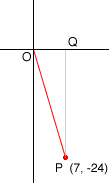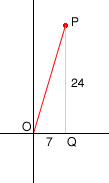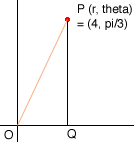Quandaries and Queries Who is asking: Parent Level: All Question: I am trying to help my son with his home, but I don't remember these conversions. Please help. 1. Convert the following coordinates Cartesian x = 7, y = -24 2. Convert the following coordinates Polar r = 4, theta = pi / 3 Hi, I do it with a diagram.In polar coordinates r is the distance |OP| and theta is the angle that the line OP makes with the positive x-axis, usually measured counterclockwise. When P is (7, -24), |OP| = sqrt(72 + 242) = 25 To find theta I looked at the diagram below.The measure of the angle POQ is tan-1(|QP|/|OQ|) = 73.74o. Thus theta, the angle QOP in the first diagram is 360o - 73.740 = 286.26o. (Maybe you want this in radians.) For the second [art again draw a diagram.Since |OP| = 4 and angle POQ is pi/3, |OQ| = 4cos(pi/3) = 2 and |QP = 4sin(pi/3) = 2sqrt(3). Penny Go to Math Central